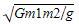Courses

# Test: Law Of Gravitation

## 10 Questions MCQ Test Physics Class 11 | Test: Law Of Gravitation

Description
This mock test of Test: Law Of Gravitation for Class 11 helps you for every Class 11 entrance exam. This contains 10 Multiple Choice Questions for Class 11 Test: Law Of Gravitation (mcq) to study with solutions a complete question bank. The solved questions answers in this Test: Law Of Gravitation quiz give you a good mix of easy questions and tough questions. Class 11 students definitely take this Test: Law Of Gravitation exercise for a better result in the exam. You can find other Test: Law Of Gravitation extra questions, long questions & short questions for Class 11 on EduRev as well by searching above.
QUESTION: 1

### SI unit of gravitational constant is __________.

Solution:

We know that F = Gm1m2 / R2
Thus we get [G] = [F.R2 / m2 ]
= [F] x L2 x M-2
= [F] M-2 L2
= N kg-2 m2

QUESTION: 2

### The dimensions of gravitational constant G is

Solution:

From Newton’s law of gravitation,

Force (F) = [GmM] × r-2

Gravitational Constant (G) = F × r2 × [Mm]-1  . . . . (1)

Since, Force (F) = Mass × Acceleration = M × [LT-2]

∴ The dimensional formula of force = M1 L1 T-2 . . . . (2)

On substituting equation (2) in equation (1) we get,

Gravitational Constant (G) = F × r2 × [Mm]-1

Or, G = [M1 L1 T-2] × [L]2 × [M]-2 = [M-1 L3 T-2].

Therefore, the gravitational constant is dimensionally represented as M-1 LT-2.

QUESTION: 3

### The mass of the body on moon is 40kg, what is the weight on the earth.

Solution:

We know that,

Weight = Mass * Accelertion due to gravity

So, Wt. = 40*9.8=392 Newtons

QUESTION: 4

The tides are formed due to:

Solution:

Tides refer to the rise and fall of our oceans' surfaces. It is caused by the attractive forces of the Moon and Sun's gravitational fields as well as the centrifugal force due to the Earth's spin.

QUESTION: 5

Two bodies with same mass “m” separated by a distance “r” exert a gravitational force of F on each other. Suppose the distance between them is doubled and the force becomes F’. The ratio of two forces is

Solution:

We know that the force of gravitation is inversely proportional to square of the distance between the two bodies,

i.e. F∝ r-2

Hence, when the distance between them will be doubled, the force will be reduced by 4 times

So, the ratio will be 4:1

QUESTION: 6

What is the value of G in cgs system of units?

Solution:

The value of G is universally constant.

6.67 × 10^-8 dyne.cm²/g²

we know ,
1 dyne = 10^-5 N
1 cm = 10^-2 m
1 g = 10^-3 kg
use this here ,

6.67 × 10^-8 × ( 10^-5) .(10^-2)²/(10^-3)²

= 6.67 × 10^-8 { 10^-5× 10^-4 /10^-6} Nm²/kg²

= 6.67 × 10^-8- × 10^-3 N.m²/kg²

= 6.67× 10^-11 Nm²/kg²

QUESTION: 7

Gravitation follows:

Solution:

Yes, Gravitational force strictly follows Newton’s Third Law of motion. You can think of it since both were formulated and discovered by Sir Isaac Newton.

Newton’s 3rd Law states that for every action force there is an equal and opposite reaction force. This too applies to gravitation.

For instance, we take a cricket ball and earth. Comparing their sizes we find that there is a lot difference between their sizes. Also earth has much greater mass than the ball. You should that gravitational force depends on mass. A heavier object exerts much greater gravitational force than a lighter object.

So when a ball hits the earth, the earth applies an equal and opposite force to the ball. so the ball bounces back.

When one body exerts a force on a second body, the second body simultaneously exerts a force equal in magnitude and opposite in direction on the first body. ... A fourth law is often also described in the bibliography, which states that forces add up like vectors, that is, that forces obey the principle of superposition.

QUESTION: 8

G is a universal gravitational constant, its value is

Solution:

We know that [g] = [G.m.m / r.r]
That is unit of G must be same as unit of g.r.r / m.m
Which is Nm2 /kg2
Also we know that G is very very less than 1 as the gravitational force is a very weak force.

QUESTION: 9

In orbit, objects inside a spacecraft feel weightless

Solution:

Astronauts float around in space because there is no gravity in space. Everyone knows that the farther you get from Earth, the less the gravitational force is. Well, astronauts are so far from the Earth that gravity is so small. This is why NASA calls it microgravity.

QUESTION: 10

What pulls a ball back to earth?

Solution:

We know that g = Gm1m2/r2
We have G = 6.672
M1 = 12kg
M2  = 4kg
g = 14N
Hence we get r =Which comes out to be 1.43 x 10-5